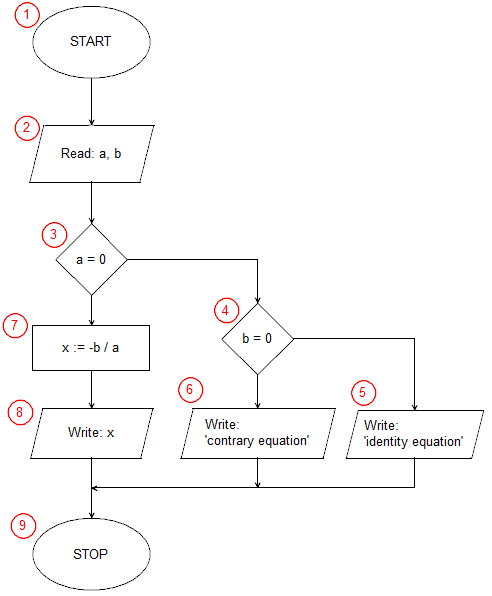# Implementation of a linear equation solving algorithm in C ++```#include <iostream>
using namespace std;
int main(void){
float a, b;
cout<<"a = ";
cin>>a;
cout<<"b = ";
cin>>b;
if(a==0)
if(b==0)
cout<<"identity equation";
else
cout<<"contrary equation";
else{
float x;
x=-b/a;
cout<<"x = "<<x;
};
cout<<endl;
char c;
cin>>c;
}```

In the first line of the program, we attach the iostream header file (input-output streams). This is necessary because in the further part of the program you will want to be able to write on the screen (cout) and load input data from the keyboard (cin). In the second line, we inform the compiler that we will use in the program names defined in the std namespace (they will be: cin, cout, endl), if we did not, it would be necessary to use a longer record of qualified names (std :: cin, std :: cout, std :: endl). In C++, the program starts with the main function. In our example, this is the only function of the program. Line 3 contains the beginning of the main function definition, this is the beginning of the implementation of our algorithm (block START (1)). In the next line, we declare two variables a, b, which we will be using in a moment. In C++ variables can be declared anywhere before they are used, therefore we do not declare an x ​​variable here immediately, which we may not need at all.

The lines 5 to 8 perform the input/output operation (2) in which the input data is taken - the coefficients a and b of the equation.

The conditional statement from line 9 is the selection block (3) from our algorithm. The instruction checks if the coefficient a is equal to 0. When the condition is satisfied, the instructions included in lines 10 to 14 are implemented (in the algorithm block selection (4) and input-output blocks (5) and (6)). When the condition is not satisfied, the statement after the else keyword is executed, in our case it is a compound statement, enclosed in logical brackets {} between lines 14 and 18. First, we declare the variable x on line 15, now we know that we will needed, then in line 16 we calculate the solution of the equation x = -b / a (operational block (7)), then on line 17 print the result (input-output block (8)).

Instructions in lines 19 - 21 have no relation to the implementation of the our algorithm. They are used to stop the program until entering any character and pressing the enter key, which prevents the program window from closing immediately.

The curly brace } from line 22 completes the main function and also the operation of the entire program (END block of the algorithm (9)).